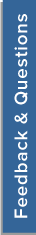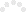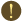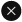We use cookies to give you the best experience on our website. If you continue to browse, then you agree to our privacy policy and cookie policy. (Last updated on: November 16, 2018).
Unfortunately, activation email could not send to your email. Please try again.# Better Axis Number Formatting#### Replies:

78658 Dec 22,2008 03:56 PM UTC Dec 26,2008 12:26 PM UTC WinForms 1
 Subscribe to this postTags: ChartRobert Avery
Asked On December 22, 2008 03:56 PM UTC

I have found the default number formatting of the xy axes in SyncFusion charts not very good... an alternative is below, but I know there are certain number ranges that still look a little funny in my code.

Public Function MakeNiceRange(ByVal min As Double, ByVal max As Double, ByVal rangePaddingType As Syncfusion.Windows.Forms.Chart.ChartAxisRangePaddingType) As Syncfusion.Windows.Forms.Chart.MinMaxInfo Implements Syncfusion.Windows.Forms.Chart.INiceRangeMaker.MakeNiceRange
Dim lngRangeMin As Double
Dim lngRangeMax As Double
Dim dbl As Double

Try
If min > max Then
min = 0
max = 1
dbl = 0.2
Else

If _ForceZero Then
lngRangeMin = 0
Else
lngRangeMin = min
If PreferZero Then
If lngRangeMin > 0 Then
If min < 1.5 * (max - min) Then
lngRangeMin = 0
End If
End If
End If
End If
Debug.Assert(_DesiredIntervals > 0)

dbl = (1.2 * (max - lngRangeMin)) / _DesiredIntervals
Dim factor As Double = 10 ^ (Math.Floor(Math.Log10(dbl)) - 1)
dbl = Math.Round(dbl / factor) * factor
'dbl = Math.Round(dbl / 500000) * 500000
'Math.Round(dbl, 1, MidpointRounding.ToEven)
lngRangeMax = Math.Ceiling(max / dbl) * dbl
If lngRangeMin <> 0 Then lngRangeMin = Math.Floor(min / dbl) * dbl

'Debug.Print("Calcing MinMaxInfo for {max = " & max & ", min = " & min & "} = {min = " & lngRangeMin & ", max = " & lngRangeMax & ", int = " & dbl & "}")

If lngRangeMax = lngRangeMin Then
lngRangeMax = lngRangeMax + 1
End If
End If

Return New MinMaxInfo(lngRangeMin, lngRangeMax, dbl)
Catch ex As Exception
Debug.Assert(False)

Return Nothing
End Try
End Function
Private Function HumanReadableRound(ByVal Value As Double) As Double

Try

Dim factor As Double = 10 ^ (Math.Floor(Math.Log10(Value)) - 1)
Dim d As Double = Value / factor

Dim adbl() As Double = {10, 15, 20, 25, 50, 100}
Dim min As Double = 999999999
Dim v As Integer

min = Math.Abs(adbl(0) - d)
For i As Integer = 1 To adbl.Count - 1
If min > Math.Abs(d - adbl(i)) Then
min = Math.Abs(adbl(i) - d)
v = i
End If
Next

Return adbl(v) * factor
Catch ex As Exception

End Try
End FunctionManohari [Syncfusion]
Replied On December 26, 2008 12:26 PM UTC

Hi Robert,

Thanks for your feedback and the valuable suggestion. I will forward this to our team and we will consider this in our future releases.

Thanks for your interest in Syncfusion products.

Regards,
Manohari.R

CONFIRMATION

This post will be permanently deleted. Are you sure you want to continue?

Sorry, An error occured while processing your request. Please try again later.You are using an outdated version of Internet Explorer that may not display all features of this and other websites. Upgrade to Internet Explorer 8 or newer for a better experience.